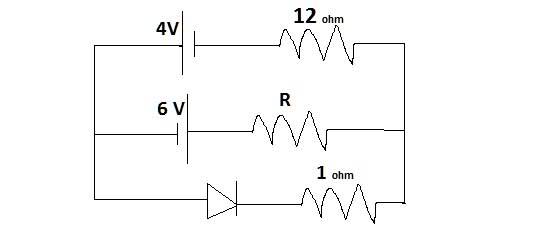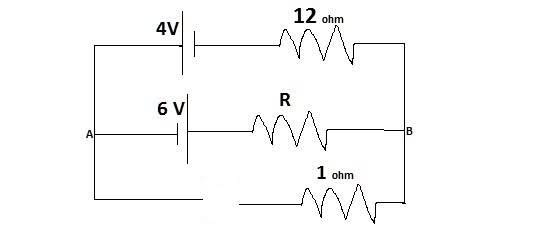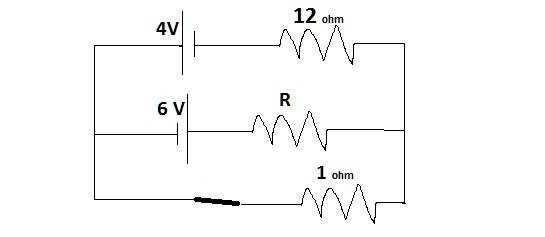# Physics P-n Junction Diode Problem

Q:-Find the current through the resistance R in the figure:-, if the value of R is:-
a.12Ω
b.48Ω.
Assume resistance offered by diode is 0 in forward bias and ∞ in reverse bias.

Ohms Law:-
V=Ri

## The Attempt at a Solution

I tried using kirchoff's law but we don't know its in reverse bias or forward.
Then I imagined the circuit without the diode wire so as to calculate the potential drop b/w the ends(this would tell whether its Forward bias or reverse) but realized that resistance would change if we connect the wire again? Plz help!

## Answers and Replies

gneill
Mentor
Hi Avi1995, welcome to Physics Forums.

You had a good idea, calculating the voltage that would appear across the open terminals of the diode location.

If you remove the diode from the circuit and find the potential that would appear at its open terminals you can determine whether the diode should be considered to be forward or reverse biased. Once you know that you can replace the diode with the appropriate open circuit or short circuit and proceed to find the current through R.

So, what are the open circuit voltages that appear for each case (be sure to specify the polarity!).

Sorry for the delay but here is my attempt:-
a)R=12Ω
After removing the diode and applying kirchoff's law:-
-10+12i+12i=0
24i=10
i=5/12=0.43A
Potential drop btw A and B is:-Vb-Va=6-5=1v
Hence in reverse bias we can disconnect the diode ans is 0.43A, WHICH IS CORRECT!
b)R=48Ω
Proceeding same as before we get
:-
i(without the diode)=1/6
Vb-Va=6-8=-2V
this Means Va is at higher potential hence forward bias.
Circuit is:-Solving we get(I am short of time but I solved on paper)
current through resistor is
i=0.13A Correct!
Thanks very much but I have one last ques.
If we remove the diode, what about the 1 Ω resistor, wont it cause any change in potential drop, is it safe removing the diode?

gneill
Mentor
If we remove the diode, what about the 1 Ω resistor, wont it cause any change in potential drop, is it safe removing the diode?

If no current flows through a resistor then there is no potential drop across it. By removing the diode an open circuit results, so no current can flow. Thus the "diode end" of the resistor must be at the same potential as its other end, namely at the same potential as your node B.